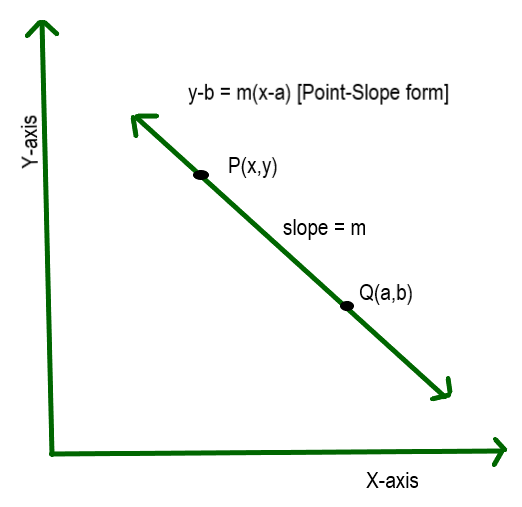# Point Slope Form Formula of a Line

In geometry, there are several forms to represent the equation of a straight line on the two-dimensional coordinate plane. There can be infinite lines with a given slope, but when we specify that the line passes through a given point then we get a unique straight line. Different forms of equations of straight line are:

• Slope-Intercept form
• Point-Slope form
• Standard form
• Intercept form
• Vertical form
• Horizontal form.

So point-slope form is the form of the equation of the straight line which uses a point and the slope of the line to form the equation of the straight line. There exist many straight lines with the same slope but the straight line passing through that point is unique and the equation is also unique. In this article let us see how to form the equation of the straight line using the point-slope form in the 2D plane.

Equation of a straight line if the point on the line is (a, b) and the slope of the line is m.

y – b = m(x – a)Point-Slope form

Derivation of the above formula:

We know that slope is defined as slope = difference in y co-ords/ difference in x co-ords

So, for the points P(x,y) and Q(a,b) the slope is m = (y – b)/(x – a)

=> m(x – a) = y – b

=> y – b = m(x – a)

Where (x,y) is the points that satisfy the equation and lie on that line.

It can be further extended to 2 point slope form if two points are given let two points be (x1, y1) and (x2, y2) then two point slope form is

Slope is given as m = (y2 – y1)/(x2 – x1)

Substituting m in one point slope form considering (x1,y1) as a point:

y – y1 = (y2 – y1)/(x2 – x1) * (x – x1)

### Sample Problems

Question1: Find the slope of the line that passes through (5,3), (6,4).

Solution:

The slope of the line when two points are given is m = y2 – y1/x2 – x1

= 4 – 3/6 – 5

= 1/1 =1

So slope of the line is 1

Question 2: Find the slope of the line when the equation of the line is 2x – 3y + 1.

Solution:

The slope of the line when equation of line is given in the form of ax + by + c is -a/b

a = 2, b = -3

m = -(2/-3)

So slope of the line is 2/3

Question 3: Find the equation of the line using point-slope form whose slope is 5 and point is (2,5)

Solution:

Given m = 5, (a,b) = (2,5)

So using point-slope form : y-b = m(x-a)

=> y-5 = 5* (x-2)

=>y -5 = 5x-10

=>5x-y-5 = 0

Equation of the line is 5x – y – 5 = 0

Question 4: Find the equation of the line using point-slope form whose slope is 2 and point is (1,3)

Solution:

Given m = 2 , (a,b) = (1,3)

So using point-slope form : y-b = m(x-a)

=> y-3 = 2* (x-1)

=>y -3 = 2x-2

=>2x-y+1 = 0

So the equation of the line is 2x – y + 1 = 0

Question 5: Find the equation of the line using point-slope form whose slope is 8 and point is (4,3)

Solution:

Given m = 8, (a,b) = (4,3)

So using point-slope form : y-b = m(x-a)

=> y-3 = 8 (x-4)

=> y – 3 = 8x – 34

=> 8x -y -31 = 0

Equation of the line is 8x – y -31 = 0

Question 6: Find the equation of the line passing through 2 points (3,6) and (4,8).

Solution:

Since 2 points are given 2 point slope form can be used:  y-y1 = (y2-y1)/(x2-x1) * (x-x1)

Given x1 = 3, y1 = 6, x2 = 4, y2 = 8

2 point slope form: y-y1 = (y2-y1)/(x2-x1) * (x-x1)

=> y -6 = (8-6 )/(4-3) * (x-3)

=> y-6 = 2*(x-3)

=> y -6 = 2x-6

=> 2x-y =0

So equation of the line is 2x-y = 0

Question 7:  Find the equation of the line passing through 2 points (1,5) and (4,17).

Solution:

Given x1 = 1, y1 = 5,  x2 = 4, y2 = 17

2 point slope form : y-y1 = (y2-y1)/(x2-x1) * (x-x1)

=> y – 5 = (17-5)/(4-1) * (x-1)

=>y-5 = 3 * (x-1)

=>y -5 = 3x -3

=> 3x – y + 2 = 0

So equation of the line is 3x – y + 2 = 0

Whether you're preparing for your first job interview or aiming to upskill in this ever-evolving tech landscape, GeeksforGeeks Courses are your key to success. We provide top-quality content at affordable prices, all geared towards accelerating your growth in a time-bound manner. Join the millions we've already empowered, and we're here to do the same for you. Don't miss out - check it out now!

Previous
Next